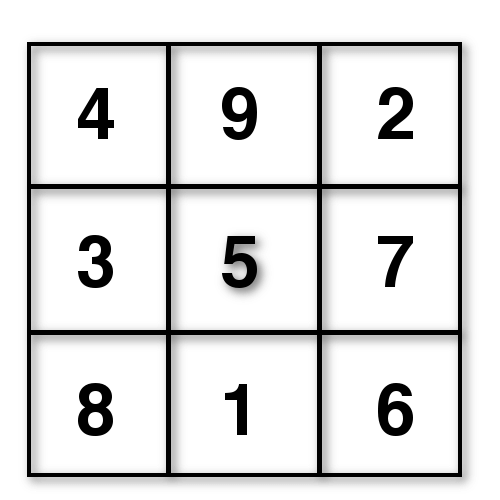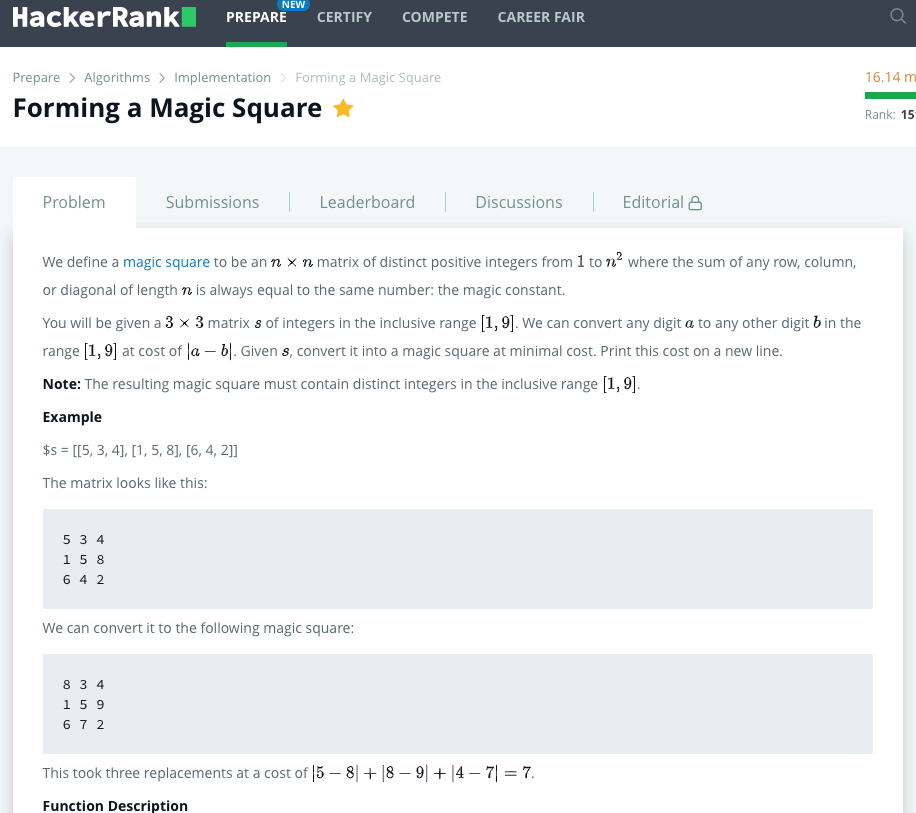# Magic Squares in JavaScriptIn mathematics, a “magic square” is a matrix of numbers where each row, column, and diagonal add-up to the same number. That number is called the “magic constant“. The integers used are only positive, and do not repeat. The constant sum is determined by the size of the square and is described by a formula:

```M = n * ((n^2 + 1) / 2)
```

Facts about the properties and classifications of these numeric formations have been discussed by scholars for millennia. Knowledge of this topic goes back thousands of years, and can be found referenced throughout the world.

## History & Culture

Magic squares have a fascinating historical and cultural significance, often with mystical undertones. They are mentioned in the I Ching, Brhat Samhita, and other works concerned with the transcendental and occult. They can be seen used in art, divination, perfumery, recreational gaming, computer science, and more.In the Brhat Samhita, the magic square is used as a symbolic representation of the planets. It makes use of magic squares in the creation of talismans for astrological purposes.

For the purposes of this blog post, we’ll view them through the lens of software engineering.

## 3×3 Magic Squares

There’s so much to cover on this topic. I’ll narrow it down to 3×3 lattices (magic squares can actually be any size), specifically in the context of the JavaScript programming language. A quad of numbers, like the one pictured above, can be described as a two-dimensional array:

```const magicSquare = [[4, 9, 2], [3, 5, 7], [8, 1, 6]];
```

Squares of this size always have a magic constant of 15. And, the number 5 will be in the middle. There’s additional logic that explains which numerals can appear where and why. Those ideas are explored in the comments section of a HackerRank coding challenge titled “Forming a Magic Square”.

## HackerRank Coding Challenge

This coding problem found on HackerRank asks programmers to figure out what it would take to convert a 3×3 matrix of integers into a magic square. The input array is almost valid, but requires a few replacements. For each change, we must track the difference between the numbers and return the total variance – referred to as the “cost”. The correct answer will be the lowest cost required to convert the input data into a magic square.The difficulty of this assignment is considered “medium”. The first step is realizing that there are a finite number of valid magic square configurations. As it turns out, there are exactly eight 3×3 permutations:

```const magicSquares = [[[4, 9, 2], [3, 5, 7], [8, 1, 6]],
[[6, 1, 8], [7, 5, 3], [2, 9, 4]],
[[8, 1, 6], [3, 5, 7], [4, 9, 2]],
[[2, 9, 4], [7, 5, 3], [6, 1, 8]],
[[8, 3, 4], [1, 5, 9], [6, 7, 2]],
[[4, 3, 8], [9, 5, 1], [2, 7, 6]],
[[6, 7, 2], [1, 5, 9], [8, 3, 4]],
[[2, 7, 6], [9, 5, 1], [4, 3, 8]]];
```

Starting with any one of these, we can generate the other seven programmatically. The subsequent arrangements can be derived through rotation and reflection. Using JavaScript, I rotate an initial seed square three times to have the first four series. Then, I flip each one of those to get the final records.

```function generateMagicSquares(magicSquare1){
const magicSquares = [];
magicSquares.push(magicSquare1);

// we need to rotate it 3 times to get all rotations
for(let i = 0; i < 3; i++){
var rotation = magicSquares[i].map((val, index) => magicSquares[i].map(row => row[index]).reverse());
// console.log(rotation)
magicSquares.push(rotation);
}

// and then flip each one
for(let i = 0; i < 4; i++){
var flipped = magicSquares[i].map((_, colIndex) => magicSquares[i].map(row => row[colIndex]));
magicSquares.push(flipped);
}

return magicSquares;
}

const magicSquare1 = [[4, 9, 2], [3, 5, 7], [8, 1, 6]];
const magicSquares = generateMagicSquares(magicSquare1);
console.log(magicSquares);```

To solve this exercise, we’ll take the input array and compare it to each of the valid magic squares. We keep track of the cost on each iteration, and finally return the minimum.

```function formingMagicSquare(s){
const magicSquares = [[[4, 9, 2], [3, 5, 7], [8, 1, 6]], [[6, 1, 8], [7, 5, 3], [2, 9, 4]], [[8, 1, 6], [3, 5, 7], [4, 9, 2]], [[2, 9, 4], [7, 5, 3], [6, 1, 8]], [[8, 3, 4], [1, 5, 9], [6, 7, 2]], [[4, 3, 8], [9, 5, 1], [2, 7, 6]], [[6, 7, 2], [1, 5, 9], [8, 3, 4]], [[2, 7, 6], [9, 5, 1], [4, 3, 8]]];

// let minCost = 100000;
let minCost = Number.MAX_SAFE_INTEGER;
let cost = 0;
for(let i = 0; i < magicSquares.length; i++){
cost = determineCost(s, magicSquares[i]);
if(cost < minCost){
minCost = cost;
}
}
return minCost;
}
```

You’ll notice that the initial minimum cost is set to a very high number. As I loop through each of the valid magic squares, I check if the cost is lower than the current minimum and then replace the value.

With each comparison, subtraction is used to determine the cost to complete the transformation. That code loops through each digit of each row on both 2D arrays. The absolute value of the difference between each coordinate is tallied and returned.

```function determineCost(inputArray, validMagicSquare){
let cost = 0;
for(let i = 0; i < 3; i++){ // each row

for(let j = 0; j < 3; j++){ // each digit

cost += Math.abs(inputArray[i][j] - validMagicSquare[i][j]);
}
}
return cost;
}
```

The working code all stitched together looks like this:

```<script>

function generateMagicSquares(magicSquare1){
const magicSquares = [];
magicSquares.push(magicSquare1);

// we need to rotate it 3 times to get all rotations
for(let i = 0; i < 3; i++){
var rotation = magicSquares[i].map((val, index) => magicSquares[i].map(row => row[index]).reverse());
// console.log(rotation)
magicSquares.push(rotation);
}

// and then flip each one
for(let i = 0; i < 4; i++){
var flipped = magicSquares[i].map((_, colIndex) => magicSquares[i].map(row => row[colIndex]));
magicSquares.push(flipped);
}

return magicSquares;
}

function determineCost(inputArray, validMagicSquare){
let cost = 0;

for(let i = 0; i < 3; i++){ // each row

for(let j = 0; j < 3; j++){ // each digit

cost += Math.abs(inputArray[i][j] - validMagicSquare[i][j]);
}
}

return cost;

}

function formingMagicSquare(s){
// const magicSquares = [[[4, 9, 2], [3, 5, 7], [8, 1, 6]], [[6, 1, 8], [7, 5, 3], [2, 9, 4]], [[8, 1, 6], [3, 5, 7], [4, 9, 2]], [[2, 9, 4], [7, 5, 3], [6, 1, 8]], [[8, 3, 4], [1, 5, 9], [6, 7, 2]], [[4, 3, 8], [9, 5, 1], [2, 7, 6]], [[6, 7, 2], [1, 5, 9], [8, 3, 4]], [[2, 7, 6], [9, 5, 1], [4, 3, 8]]];
const magicSquare1 = [[4, 9, 2], [3, 5, 7], [8, 1, 6]];
const magicSquares = generateMagicSquares(magicSquare1);

// let minCost = 100000;
let minCost = Number.MAX_SAFE_INTEGER;
let cost = 0;
for(let i = 0; i < magicSquares.length; i++){
cost = determineCost(s, magicSquares[i]);
if(cost < minCost){
minCost = cost;
}
}
return minCost;
}

const finalCost = formingMagicSquare([[4, 9, 2], [3, 5, 7], [8, 1, 5]]);
console.log(finalCost);
</script>
```

This solution was not intuitive to me and took some research and experimentation. It was interesting to learn about the concept of magic squares (and other shapes) along the way.